# Multiple Choice questions on Electrostatics

In this page we have Multiple Choice questions on Electric Charge for Jee Main and Advanced . Hope you like them and do not forget to like , social share and comment at the end of the page.
Question 1
A charge of magnitude q is divided into two parts such that force between resulting two charges is maximum when separated through some distance r. The division of charges would be
(a) 3q/8 , 5q/8
(b) 2q/4 , 2q/4
(c) q/2 , q/2
(d) 3q/6 , 3q/6

Question 2
Consider a system of three charges q/3, q/3 and –2q/3 placed at points A, B and C respectively as shown in the figure. Take O to be the centre of the circle of radius R and angle CAB = 60º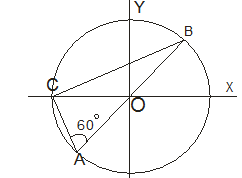(a) The electric field at point O is q/8πε0R2
(b) The magnitude of the force between the charges at C and B is q2/54πε0R2
(c) The potential energy of the system is zero
(d) The potential at point O is q/12πε0R

Question 3
Four charges q, 2q, 3q, 4q are placed at corners A, B, C and D of a square as shown below in the figure. The field at centre O of square has the direction along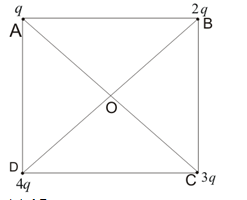(a) AB
(b) CB
(c) AC
(d) DB

Question 4
A point charge q is placed at geometrical centre of one of the face of a cube. The total flux through the cubical surface due to charge is
(a)$\frac {q}{\epsilon _0}$
(b) $\frac {q}{2\epsilon _0}$
(c) $\frac {2q}{\epsilon _0}$
(d) 0

Question 5
Two large metal sheets having surface charge density +σ and –σ are kept parallel to each other at a small separation distance d. The electric field at any point in the region between the plates is
(a) σ/ε0
(b) σ/2ε0
(c) 2σ/ε0
(d) σ/4ε0

Question 6
A rod lies along the x-axis with one end at the origin and other at x->∞ it caries a uniform charge λ C/m. Find the electric field at the point x=-a on the x-axis
(a) $\frac {-\lambda}{4 \pi \epsilon _0 a} \boldsymbol{i}$
(b) $\frac {-\lambda}{4 \pi \epsilon _0 a^2} \boldsymbol{i}$
(c) $\frac {\lambda}{4 \pi \epsilon _0 a} \boldsymbol{i}$
(d) $\frac {\lambda}{4 \pi \epsilon _0 a^2} \boldsymbol{i}$

Question 7
Twelve charges of charge q are situated at the corners of the 12 sided polygon of side a. What is the net force on the charge Q at the centre
(a) Zero
(b) 3qQ/πε0a2
(c) qQ/πε0a2
(d) None of the above

Question 8
Two positive point charge are placed at the distance a apart have sum Q. What values of the charges , coulomb force between them is maximum
(a) q1=q1=Q/2
(b) q1=3Q/4 ,q2=Q/4
(c) q1=5Q/6 ,q2=Q/6
(d) Non of the above

Question 9
A metallic shell having inner radius R1 and outer radii R2 has a point charge Q kept inside the cavity. Electric field in the region R1 < r < R2 where r is the distance from the centre is given by
(a) depends on the value of r
(b) Zero
(c) Constant and nonzero everywhere
(d) None of the above

Question 10
Consider two statements
(A) The force with which two charges interact is not changed by the presence of the other charges
(B) Electric force experienced by the charge particle due to number of fixed point charges is vector resultant of the forces experience due to individual charges
(a) A and B both are correct
(b) A is correct only
(c) B is correct only
(d) A and B both are wrong

Question 11
A metallic solid sphere of radius R is given the charge Q. Which of the following statement is true then
(a) Electric field at points 0< r < R is zero
(b) Charge Q is on the outer surface of the sphere
(c) Electric field at r>R is given by Q/4πε0r2
(d) Electric field is perpendicular to the surface of the sphere

Question 12.
A simple pendulum consists of a small sphere of mass and positive charge q is suspended by the string of length L. The pendulum is placed in the electric field of strength E directed vertically downwards. Which of the following is true
(a)Time period of oscillation= $T= 2 \pi \sqrt { \frac {L}{g+qE/m}}$
(b)Time period of oscillation=$T= 2 \pi \sqrt { \frac {L}{g+qE/m}}$
(c) Tension in the string when the pendulum is at rest =$mq+qE$
(d)Tension in the string when the pendulum is at rest =$mq-qE$

Question 13
A rod lies on the x-axis with end and at x=-L and other end at x=L with uniform charge λ C/m. Which of the following is true. here $k=\frac {1}{4\pi \epsilon _0} (a).Electric field at any point (0,y) on the y-axis is given by E=(2kλL/y√(y2+L2))j (b) For point on the Y-axis greater than y>>L E=(2kλL/y2)j (c) Electric field if L->∞ E=2kλ/y (d) None of the above Question 14 A particle of mass m and charge q is thrown horizontally with a velocity v from top of the building of height H. An electric field exists in the plane and it is horizontally away from the building which of the following is true (a) Range of the particle is greater than v√(2H/g) (b) Time of flight is √(2H/g) (c) Path is parabolic (d) None of the above Question 15 At a point on the axis of an electric dipole (a) Electric field is zero (b) Electric potential is zero (c) Neither electric field nor electric potential is zero (d) Electric field is directed perpendicular to axis Question 16 A point charge (Q) is located at the centre of a cube of edge length a, find the final electric flux over one face of the cube a. Q/ε0 b. Q/6ε0 c. 6Q/ε0 d. none of the above Question 17 Three point charges q + Q, q, q - Q are enclosed by the surface S. What the net flux crosses S a. 3q b. 2q c. 3q - Q d. can not be determine based on the data given in question Question 18 Find the electric field inside the sphere which carries a charge density proportional to the distance from the origin ρ = kr a. ρ/ε0 b. ρr/ε0 c. ρr20 d. none of the above Question 19 A point charge Q(C) is placed at the origin. Find the electric flux of which an area 4π m2 on a concentric spherical shell of radius R a. Q/R2ε0 b. Q/ε0 c. Q/4R2ε0 d. none of the above Question 20 As per Gauss law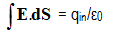Which of the following is true about this a. This is valid for symmetrical surface only b. E is the electric field to the charge inside the surface c. Electric flux on the closed surface due to outside charge is always zero d. none of the above Question 21 A uniform line charge with linear density λ lies along the y-axis. What flux crosses a spherical surface cantered at the origin with r = R a. 2Rλ/ε0 b. Rλ/ε0 c. λ/ε0 d. none of the above Linked comprehension type (A) A thin metallic wire ring of radius R and area of cross-section A carries an electric charge of q Coulombs. The centre of the ring contains a charge Q>>q with sign of Q same as that of q. Question 22 The force with which ring stretches is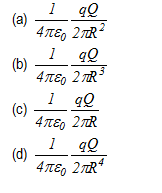Question 23 Change in radius of the ring would be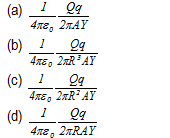where Y is the young’s modulus of the ring Linked comprehension type (B) A slab of uniform thickness 2d and uniform charge density ρ is lying between –d to d along x-axis and extends infinitely along y and z directions as shown below in the figure.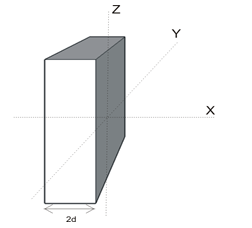Question 24 Magnitude of electric field at any point inside the slab as a function of x is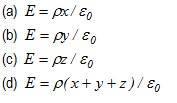Question 25 Electric field for regions outside the slab is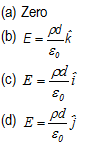Linked comprehension type (C) Question 26 A long cylinder contains charge distributed uniformly having volume charge density ρ. (A) Electric field inside this cylinder is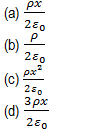Question 27 What would be the field inside the cylinder if volume charge density of the cylinder varies according to the following relation given below? ρ=kx2 where k is a constant.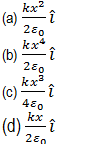Assertion reason type questions The following questions consist of two statements, Statement I and Statement II. While answering these questions choose any of the following four responses A. If both Statement I and Statement II are true and the reason is correct explanation of Statement I. B. If both Statement I and Statement II are true but reason is not a correct explanation of Statement I. C. If Statement I is true and Statement II is false. D. If both Statement I and Statement II are false. Question 28 Statement I: There is no electric field in the conductor Statement II: There are plenty of free electrons in the conductors which moves in such a way, it cancel every electric field in the conductor Question 29 Statement I: Coulombs Force is an action reaction pair Statement II: Coulombs force is non conservative in nature Question 30 Statement I: Two electric lines of force can never intersect Statement II: Electric lines of force originates from negative and terminate on positive charge Question 31 Statement I: Excess charge given to the conductor always resides on the outer surface of the conductor Statement II: electric field is zero inside the bulk material of the conductor Matrix Match question Question 32 Consider the figure given below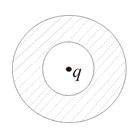A charge Q is placed inside the Spherical conductor shell Column I (A) Charge Q is at the center of the shell (B) Charge Q is displaced from center and place at distance r from the center (C) An excess charge q is given to the shell (D) A charge q is placed near the conductor shell Column II (X) Charge distribution is uniform at the inner surface of shell (Y) Charge distribution is non uniform at the inner surface of shell (Z) Charge distribution is uniform at the outer surface of shell (K) Charge distribution is non uniform at the outer surface of shell Question 33 Consider the figure given below in which an infinite conductor has uniform surface charge distribution σ0 and adjacent to it is an infinite parallel layer of charge with volume charge density ρ varying according to following relation ρ=ρ0e-αz , where z>0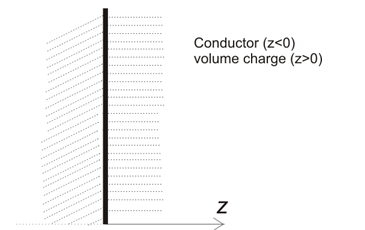Electric field due to the conductor is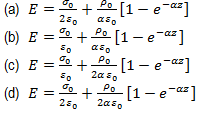Question 34 Two uniform infinite sheets of charge density +σ and –σ intersect at right angles. Electric field due to this configuration at any point in space is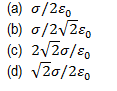Question 35 A charge Q sits at the back corner of a cube. What is the flux of electric field through the shaded surface?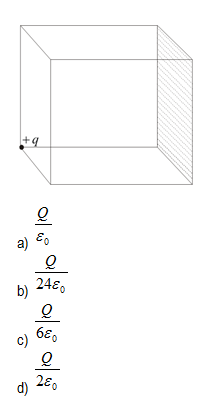Question 36 Match the column A point charge is placed at the center of a closed spherical surface. Φ : Electrical Flux through the closed spherical surface E : Electric Field through the closed spherical surface Then Mark out the correct statement Column I (A) If the point charge is displaced from the center but kept within the closed surface (B) If the point charge is displaced such that it crosses the boundary of closed surface (C) If any another charge is kept outside the surface (D) If any another charge is placed inside the surface Column II (P) Φ will change (Q) E will change (R) Φ will not change (S) E will not change Question 37 A point charge +Q is at the origin of a coordinates system . It is surrounded by a concentric uniform distribution of charge on a spherical shell at r=R for which the total charge is -2Q Which of the following is correct?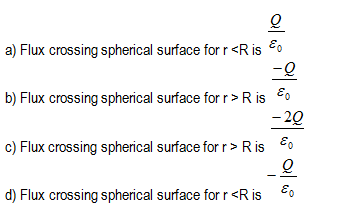Question 38 Two infinite uniform line charges of linear charge density 2 C/m lies in a XY plan at x =+a m and x=-a m.. A positive charge particle of charge q is placed at the origin and it is displaced by a distance k from origin towards +x axis. Which of the following is correct (a) The charge particle will oscillate but not harmonically for all values of 0 < k <a (b) The charge particle will oscillate harmonically for all values of 0 < k <a (c) The charge particle will oscillate harmonically for k<<<a (d) The charge particle will not oscillate Question 39 Two infinite uniform sheet of charge each with surface charge density σ are located at x=+a and x=-a. Which of the following is correct?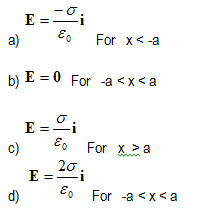Question 40 A conductor of non uniform curvature is given Q charge. Which of the following is correct (a) The charge is distributed uniformly over its volume (b) The charge is distributed uniformly over its outer surface (c) The charge has the greatest concentration on the part of greatest curvature (d) The charge has the greatest concentration on the part of least curvature Question 41 At a point on the axis of an electrical dipole (a) Electric field is zero (b) Electric potential is zero (c) Neither Electric field and electric potential is zero (d) Electric field is directed Perpendicular to the axis Question 42 A positive charge Q is uniformly distributed along a circular ring of radius R. A small test charge q is placed at the centre of the ring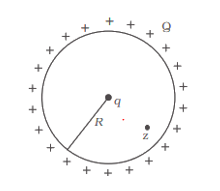Then (a) If q > 0 and is displaced away from the centre in the plane of the ring, it will be pushed back towards the centre. (b) If q < 0 and is displaced away from the centre in the plane of the ring, it will never return to the centre and will continue moving till it hits the ring. (c) If q < 0, it will perform SHM for small displacement along the axis. (d) q at the centre of the ring is in an unstable equilibrium within the plane of the ring for q > 0 Question 43 A Gaussian surface of radius R with Q at the centre is drawn in the given region of charges.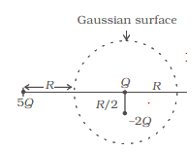Which of the following is true (a) total flux through the surface of the sphere is$ \frac {-Q}{\epsilon _0 }$(b)field on the surface of sphere due to -2Q is same everywhere (c) field on the surface of the sphere is$ \frac {-Q}{4 \pi \epsilon _0 R^2}$(d) flux through the surface of sphere due to 5Q is zero Question 44 Consider the charge configuration and spherical Gaussian surface as shown in the figure. When calculating the flux of the electric field over the spherical surface the electric field will be due to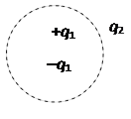(a)$q_2$(b) Only the positive charges (c) All the charges (d)$-q_1$and$+q_1\$